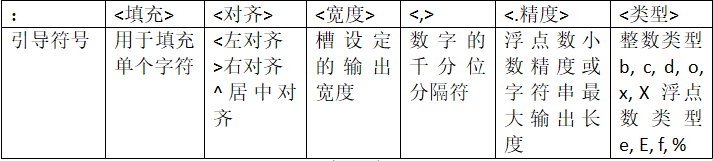# python基本数据类型

python基本数据类型

(一) 数字类型
1整数：数学中的整数

pow(x,y)函数：计算 x的y次幂
2浮点数：数学中的实数,一定带有小数部分,浮点数间运算存在不确定尾数

3复数类型：与数学中复数的概念一致
z = 1.23e-4+5.6e+89j
z.real 获得实部 z.imag 获得虚部

(二) 数值运算操作符

x + y ：x与y之和			x–y ：x与y之差
x * y ：x与y之积			x / y  ：x与y之商
x // y 取整					+ x： x本身
- x： x的负值 				x % y 余数，
x ** y幂运算，x的y次幂
x += y    x -= y     x *= y   x /= y  x //= y    x %= y    x **= y


(三) 数字类型的关系

(四) 数字运算函数
abs(x)：x的绝对值
divmod(x,y)：商余，(x//y, x%y)，同时输出商和余数
pow(x, y[, z])：幂余，(x**y)%z，[…]表示参数z可省略
round(x[, d])：四舍五入，d是保留小数位数，默认值为0
max(x1,x2, … ,xn)：最大值，返回x1,x2, … ,xn中的最大值，n不限
min(x1,x2, … ,xn)：最小值，返回x1,x2, … ,xn中的最小值，n不限
int(x)：将x变成整数，舍弃小数部分
float(x)：将x变成浮点数，增加小数部分
complex(x)：将x变成复数，增加虚数部分

(一) 字符串：由0个或多个字符组成的有序字符序列
Python字符串的编码方式：Unicode编码

(二) 字符串的使用

M缺失表示至开头，N缺失表示至结尾
<字符串>[M: N: K]，根据步长K对字符串切片

(三) 字符串的特殊字符 : 转义符

"\b"回退 "\n"换行(光标移动到下行首) “\r” 回车(光标移动到本行首)

(四) 字符串操作符
x + y 连接两个字符串x和y
n * x 或 x * n 复制n次字符串x
x in s 如果x是s的子串，返回True，否则返回False
s+t s[i] s[i:j] s[i:j:k] max(s) min(s)

(五) 字符串处理函数
len(x)：长度，返回字符串x的长度
str(x)：任意类型x所对应的字符串形式
hex(x)或 oct(x)：整数x的十六进制或八进制小写形式字符串
chr(u)： x为Unicode编码，返回其对应的字符
ord(x)： x为字符，返回其对应的Unicode编码

(六) 字符串处理方法
str.lower() 或 str.upper()：返回字符串的副本，全部字符小写/大写
str.split(sep=None)：返回一个列表，由str根据sep被分隔的部分组成
str.count(sub)：返回子串sub在str中出现的次数
str.replace(old, new)：返回字符串str副本，所有old子串被替换为new
str.center(width[,fillchar])：字符串str根据宽度width居中，fillchar可选
str.strip(chars)：从str中去掉在其左侧和右侧chars中列出的字符
str.join(iter)：在iter变量除最后元素外每个元素后增加一个str
str.index(x[,i[,j]]) 返回str从开始位置到第一次出现x的位置

(七)字符串类型的格式化：格式化是对字符串进行格式表达的方式
<模板字符串>.format(<逗号分隔的参数>)(八)格式化输出的第二种方法
a=int(input())
print(“the number is %d” % a)
print("%06d" % a) #表示输出的整数显示的位数，不足的地方用0补齐，超过六位正常表示

print(“the number is %d and %d” % (a, b))

lt = “the number is %d and %d” % (a, b)
%s: 字符串
%d: 整数
%f: 浮点型. %.2f表示小数点后显示2位
%%： 输出一个%

©️2019 CSDN 皮肤主题: 精致技术 设计师: CSDN官方博客